# 推理加速效能超越英偉達FasterTransformer 50%，開源方案打通大模型落地關鍵路徑

AI大模型推理部署的困難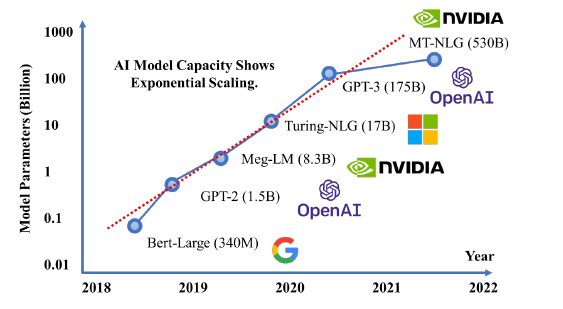模型引數的迅速增長[https://arxiv.org/abs/2111.14247]

Energon-AI系統設計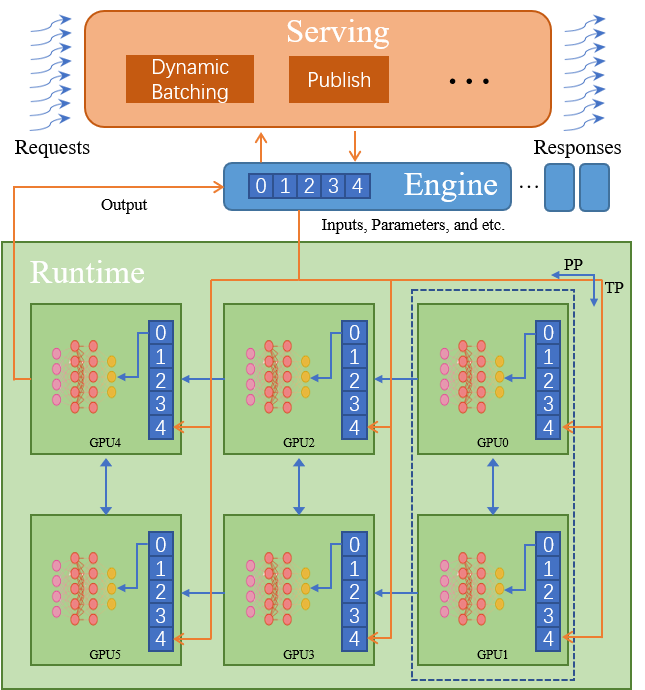Energon-AI超大模型推理系統示意圖

• Engine：單裝置推理中程式有相同的資料入口與出口，分散式訓練的主要目標是模型引數，因此無須對多個程序的輸入輸出進行管理，而多裝置推理則不同。我們希望通過良好的封裝使得Engine具有與單裝置推理完全相同的行為。我們採用了半中心化方法，主程序中使用RPC在每個裝置呼叫初始化或推理方法，使得分散式推理可以得到中心化的控制，同時每個裝置則保有自己的Tensor Parallel與Pipeline Parallel通訊邏輯。我們在每個程序中設計並維護了分散式訊息佇列，用以保證多個程序中多執行緒呼叫執行的一致性。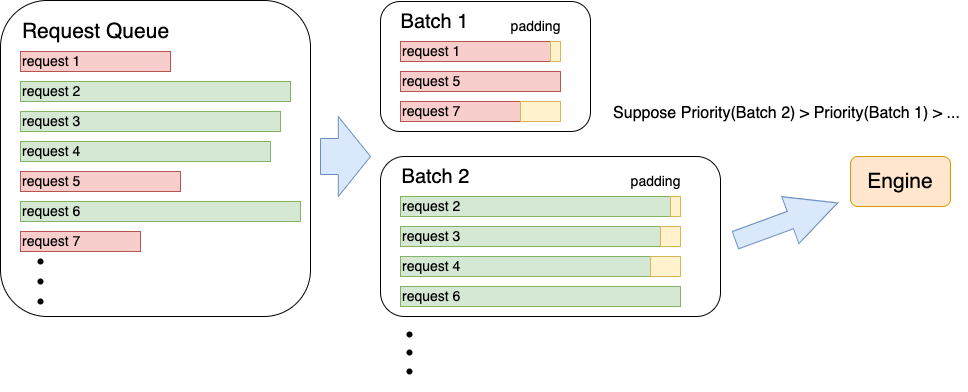Batch管理流程示意圖

## 效能測試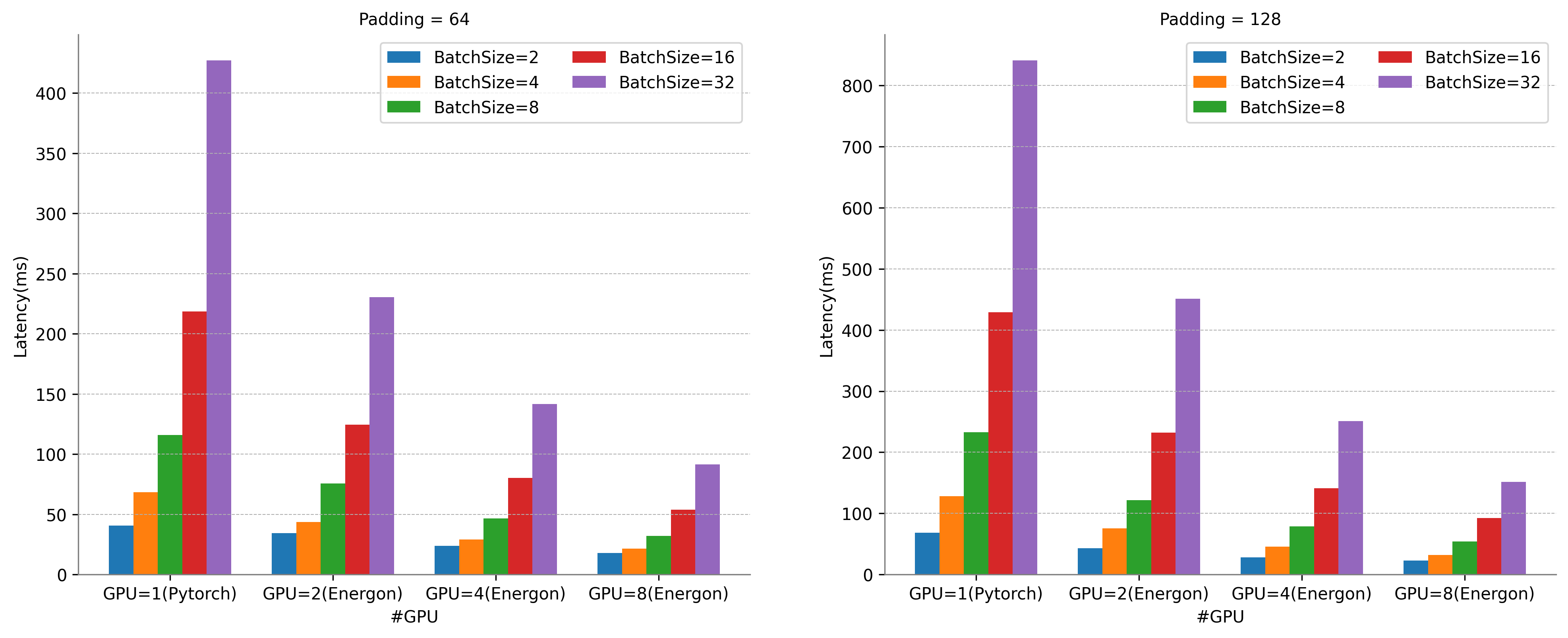Energon-AI八卡並行推理在Batch Size為32時，相比於單卡Pytorch直接推理，可獲得8.5倍的超線性加速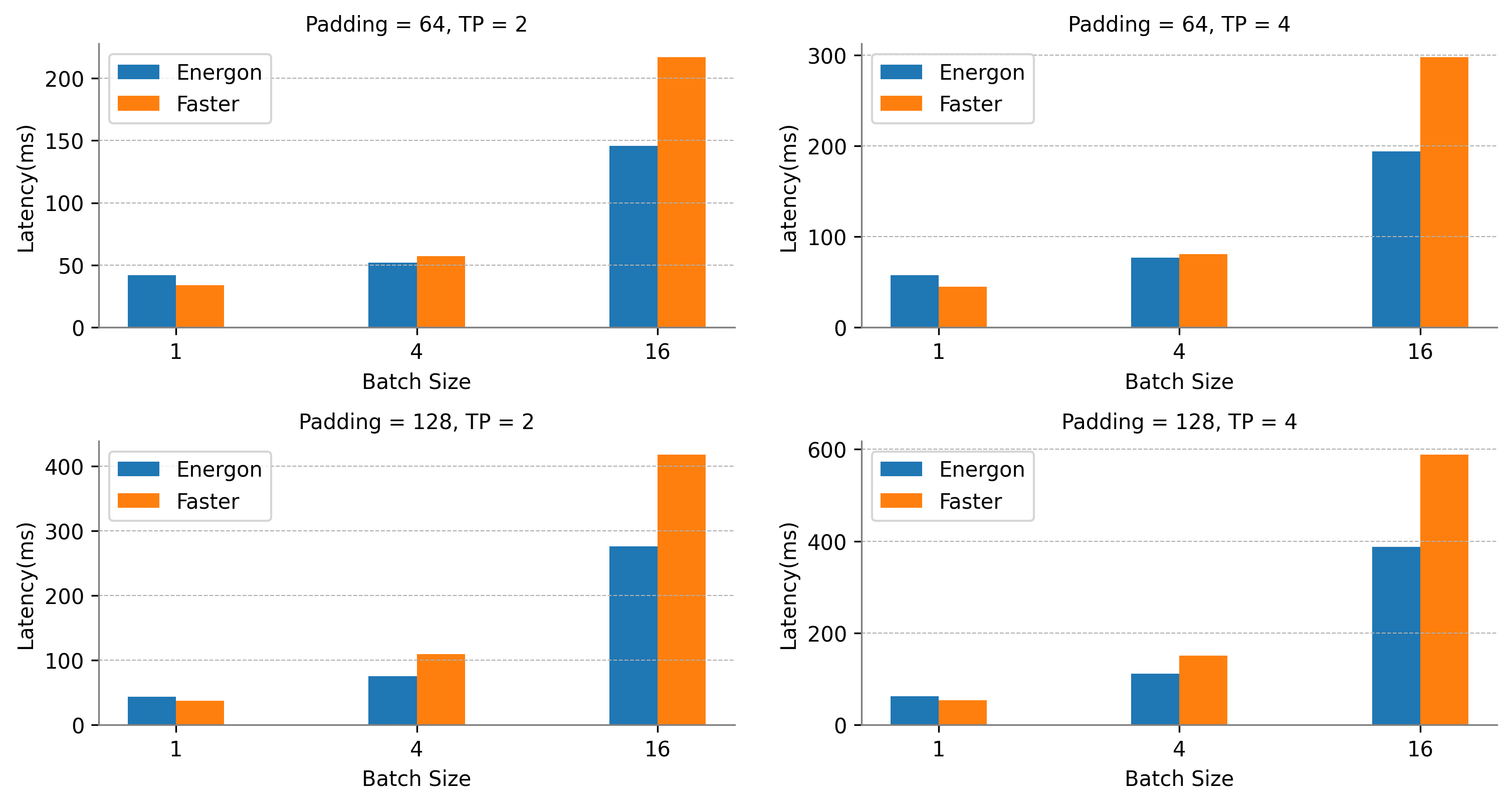Dynamic Batching吞吐量增加30%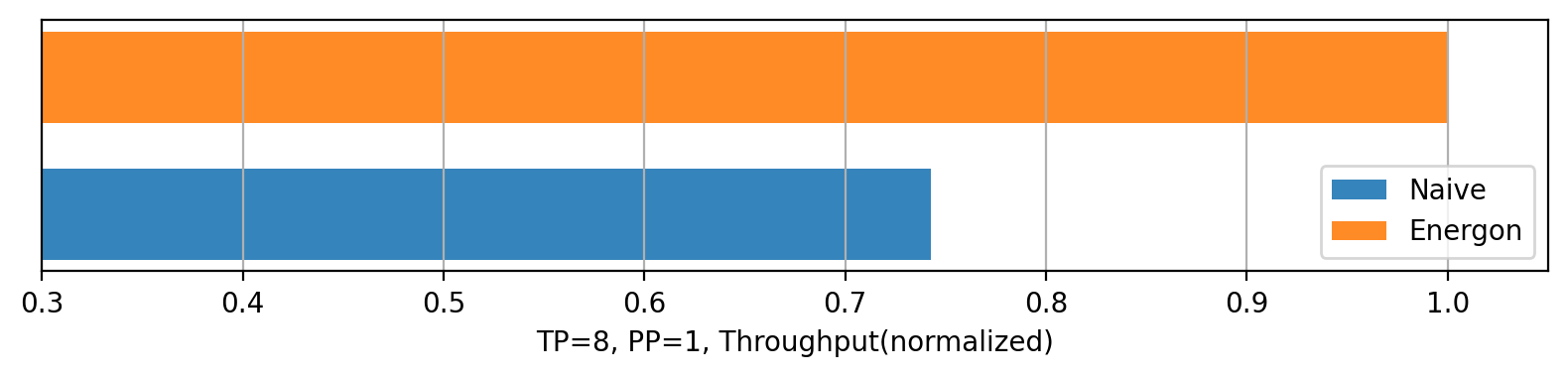## 易用性

``````from gpt import gpt3
from gpt_server import launch_engine

# for engine
model_class = gpt3
model_type = "gpt"
host = "127.0.0.1"
port = 29400
half = True
backend = "nccl"

# for parallel
tp_init_size = 4
pp_init_size = 2

# for server
engine_server = launch_engine
server_host = "127.0.0.1"
server_port = 8020
``energonai service init --config_file=gpt_config.py``

``````class MLP(nn.Module):
def __init__(self, dim, dtype, bias):
super().__init__()
self.dense_0 = Linear1D_Col(dim, dim, dtype=dtype, bias=bias, gather_output=False)
self.dense_1 = Linear1D_Row(dim, dim, dtype=dtype, bias=bias, parallel_input=True)
def forward(self, x):
x = self.dense_0(x)
x = self.dense_1(x)
return x``````

``````// Memory Allocation (only for a single paramerter).
T *d_inter_kernel = NULL
param_.ffn.intermediate_weight.kernel = d_inter_kernel;
device_malloc(&d_inter_kernel, dim * dim);

// Two MLP Layers
cublasMM_cublasLtMM_wrapper(param_.cublaslt_handle, param_.cublas_handle, CUBLAS_OP_N, CUBLAS_OP_N, n, m, k, &alpha, param_.ffn.intermediate_weight.kernel, AType_, n, attr_matmul_buf_, BType_, k, &beta, (DataType_ *)inter_matmul_buf_, CType_, n, param_.stream, cublasAlgoMap_, sm_, cublas_workspace_);

add_bias_act_kernelLauncher<DataType_>(inter_matmul_buf_, param_.ffn.intermediate_weight.bias, m, n, ActivationType::GELU, param_.stream);

n = k;

cublasMM_cublasLtMM_wrapper(param_.cublaslt_handle, param_.cublas_handle, CUBLAS_OP_N, CUBLAS_OP_N, n, m, k, &alpha, param_.ffn.output_weight.kernel, AType_, n, inter_matmul_buf_, BType_, k, &beta, (DataType_ *)(param_.transformer_out), CType_, n, param_.stream, cublasAlgoMap_, sm_, cublas_workspace_);

add_bias_input_layernorm_kernelLauncher<DataType_>(param_.transformer_out,                                                             attr_matmul_buf_, param_.ffn.output_weight.bias, param_.ffn_layernorm.gamma, param_.ffn_layernorm.beta, m, n, param_.stream);

// Communication
if(t_parallel_param_.world_size > 1)
{
all2all_gather(nccl_logits_buf_, nccl_logits_buf_, local_batch * n, t_parallel_param_, decoding_params.stream);
}``````

## 構建AI大模型生態系統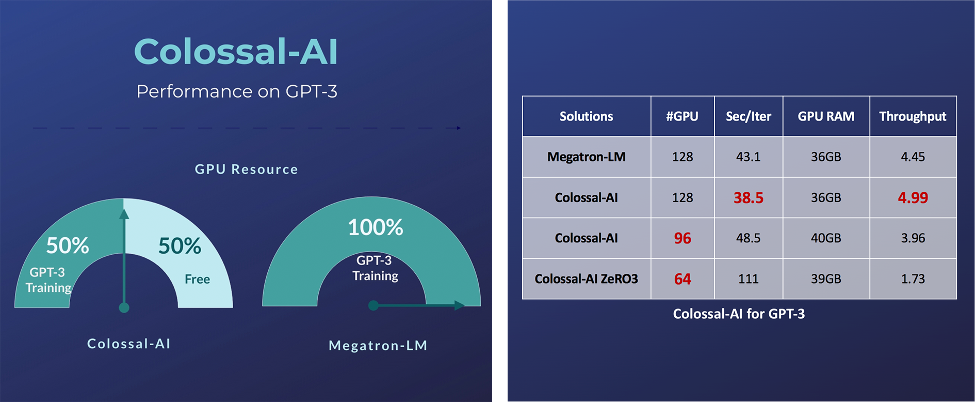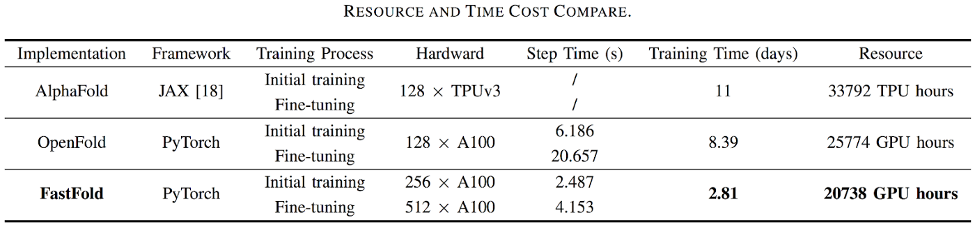Colossal-AI相容低端裝置，在僅有一塊GPU的個人PC上便能訓練高達180億引數GPT；普通的膝上型電腦，也能訓練十幾億引數的模型，相比現有主流方案，可提升引數容量十餘倍，大幅度降低了AI大模型微調和推理等下游任務和應用部署的門檻。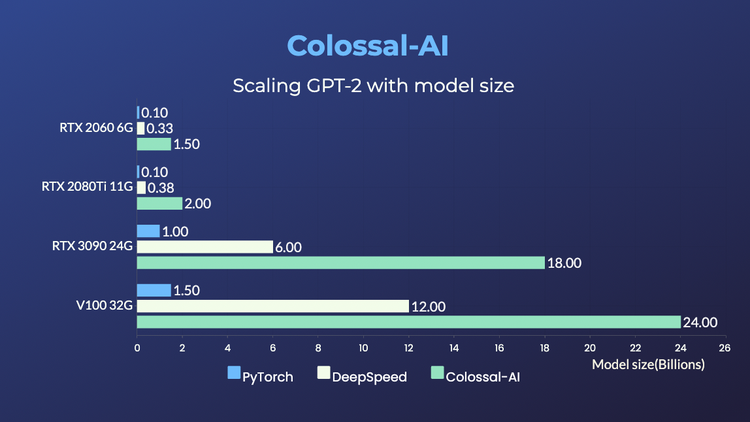Colossal-AI注重開源社群建設，提供中文教程，開放使用者社群及論壇，對於使用者反饋進行高效交流與迭代更新，不斷新增等前沿特性。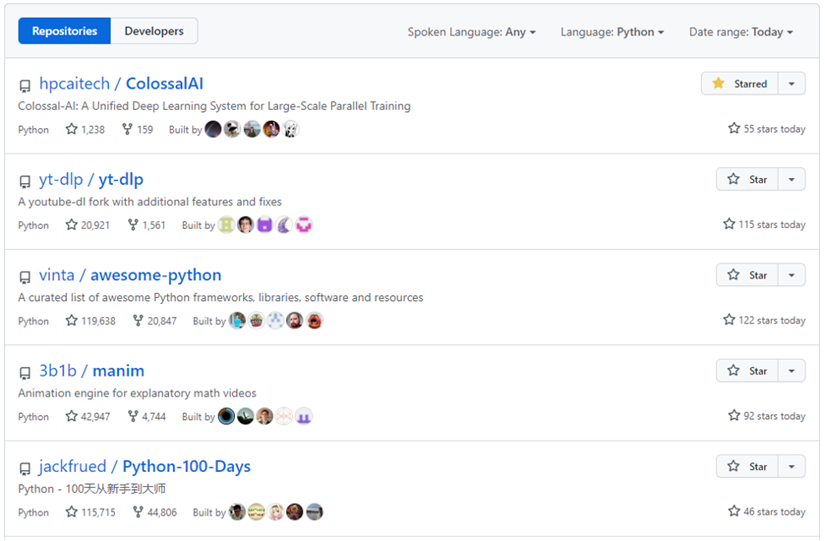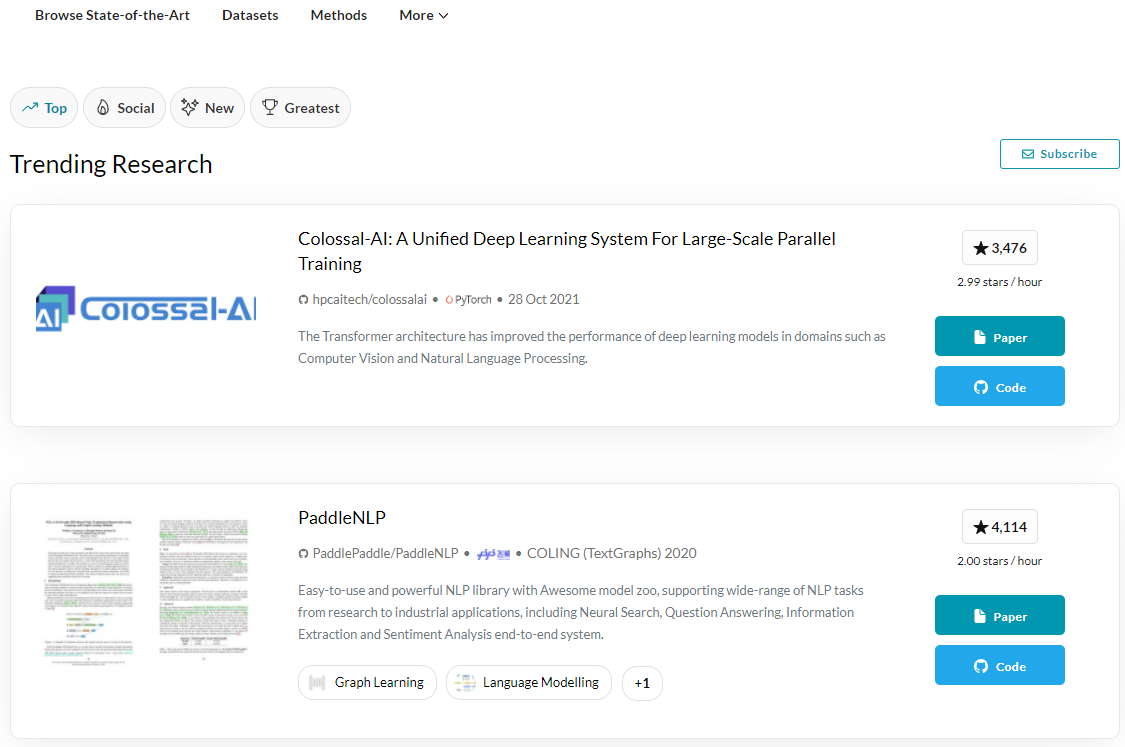## 專案團隊